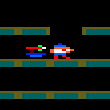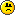### CPC Basic

Would you like to react to this message? Create an account in a few clicks or log in to continue.

# Get The Cash GameOut Bush

Posts : 11
Join date : 2019-12-02
Back in 2016 I started coding this game with CPC BASIC 3 and while I got so far into it, some bits of it appeared on CPC-Rulez and CPC-Power, but I ran into problems when I was trying to Move the Graphics down the Screen with CPC BASIC 3, which ended up in a game being completed in Hisoft Pascal.

I decided to go back to what I coded in 2016 and see if I could have the graphics moving down the screen. Earlier on I was using a whole heap of User Defined Graphics to make my Graphics look like Sprites along with some Assembly, but in this post I have replaced that with the Simple Sprite Driver I created with CPC BASIC 3.

The final result is a working game, though shortly I shall be posting a CPC BASIC 3 version of the same game using an Assembly Sprite Driver I created. The advantage with this one though is I can simply tell CPC BASIC 3 to Run in the Emulator and it'll do that.

Code:
`100 ' Get the Cash! :)110 '120 ' Use Left / Right Arrow to Get the Cash, but avoid the Obstacles130 '140 DEFWORD o, x, y, w, z : DIM objects(30)150 RANDOMIZE TIME155 MODE 0 : BORDER 0 : INK 1,26 : INK 2,13 : INK 3,25 : INK 4,2 : INK 5,11 : INK 6,6156 GOSUB 1910160 yaxis = 0170 FOR setobj=1 TO 30 STEP 3180  x1 = setobj + 1190  y1 = setobj + 2200  GOSUB 540210  objects(y1) = yaxis220  yaxis = yaxis + &A0230  obj = RND MOD 3+1240  IF (obj=1 OR obj=2) THEN objects(setobj)=1 ELSE objects(setobj)=3245  IF objects(setobj)=3 THEN p = 33 ELSE p = 1250  w = objects(x1) : z = objects(y1) : GOSUB 1050260 NEXT setobj290 xp=20 : yp=1840 : w=xp : z=yp : p=65 : exit = 0 : sc = 0 : GOSUB 1050300 FOR ys=1 TO 25 : LOCATE 13,ys : PRINT CHR\$(219);: NEXT ys310 LOCATE 14,1 : PRINT"Score:"320 LOCATE 14,2 : PRINT Sc330 WHILE exit = 0331  IF INKEY(1)=0 THEN IF xp < 44 THEN p = 97 : w = xp : z = yp : GOSUB 1050 : xp = xp + 4 : p = 65 : w = xp : z = yp : GOSUB 1050 : CALL &BD19332  IF INKEY(8)=0 THEN IF xp > 0 THEN p = 97 : w = xp : z = yp : GOSUB 1050 : xp = xp - 4 : p=65 : w = xp : z = yp : GOSUB 1050 : CALL &BD19340  FOR ps=1 TO 30 STEP 3350  x1 = ps + 1 : y1 = ps + 2360  IF objects(y1)<1840 THEN GOSUB 600 : objects(y1) = objects(y1) + &50 ELSE GOSUB 600 : GOSUB 540 : objects(y1) = 0 : objects(ps) = RND MOD 3+1365  p=65 : w = xp : z = yp : GOSUB 1050370  IF objects(ps)=1 OR objects(ps)=2 THEN p=1 : w = objects(x1) : z = objects(y1) : GOSUB 1050 ELSE p=33 : w = objects(x1) : z = objects(y1) : GOSUB 1050385  IF objects(y1)=1840 THEN IF xp = objects(x1) AND objects(ps) = 3 THEN p = 65 : w = xp : z = yp : GOSUB 1050 : sc=sc+10 : LOCATE 14,2 : PRINT sc;386  IF objects(y1)=1840 THEN IF xp = objects(x1) AND objects(ps) <> 3 THEN exit = 1390  NEXT ps450 WEND460 CLS : LOCATE 7,8 : PRINT "FINAL" : LOCATE 7,9 : PRINT "SCORE" : LOCATE 8,10 : PRINT sc470 LOCATE 8,12 : PRINT "PLAY" : LOCATE 7,13 : PRINT "AGAIN" : LOCATE 7,14 : PRINT "(Y/N)" : WHILE INKEY\$<>"" : WEND480 WHILE INKEY(46)=-1490 IF INKEY(43)<>-1 THEN GOTO 155500 WEND510 WHILE INKEY\$<>"" : WEND : CALL &BC02 : MODE 2 : END520 SOUND 135,0,0,0,2,,1 : RETURN530 ' pick random number divisible by 4540 objects(x1) = RND MOD 48550 WHILE objects(x1) MOD 4 <> 0560  objects(x1) = RND MOD 48570 WEND580 RETURN590 ' Clear Graphics600 p=97610 w=objects(x1)620 z=objects(y1)630 GOSUB 1050640 RETURN1000 ' 8x8 Sprite Driver1010 ' Entry :-1020 '  w = x Coordinate1030 '  z = y Coordinate1040 '  p = Sprite Position (1 = Hazard, 33 = Money Bag, 65 = Bat, 97 = Blank)1050 FOR y=z+0 TO z+&3800 STEP &8001060  FOR x=w+0 TO w+31070    POKE &C000+x+y,b(p)1080    p=p+11090  NEXT x1100 NEXT y1110 RETURN1900 ' Setup Graphics1910 DIM b(128):RESTORE 20101920 FOR p=1 TO 1281930  READ b(p)1940 NEXT p1950 RETURN2000 ' Hazard2010 DATA 0,0,0,02020 DATA 64,128,192,1282030 DATA 0,12,4,122040 DATA 64,128,192,1282050 DATA 0,12,4,122060 DATA 64,128,192,1282070 DATA 0,12,4,122080 DATA 0,0,0,02090 ' Money Bag2100 DATA 136,0,0,682110 DATA 100,204,204,1522120 DATA 112,224,192,1762130 DATA 112,224,240,1762140 DATA 112,192,208,1762150 DATA 112,224,240,1762160 DATA 112,192,192,1762170 DATA 16,48,48,322180 ' Bat2190 DATA 0,0,0,02200 DATA 0,0,0,02210 DATA 0,0,0,02220 DATA 0,0,0,02230 DATA 128,0,0,642240 DATA 40,0,0,202250 DATA 104,192,192,1482260 DATA 60,60,60,602270 ' Blank2280 DATA 0,0,0,02290 DATA 0,0,0,02300 DATA 0,0,0,02310 DATA 0,0,0,02320 DATA 0,0,0,02330 DATA 0,0,0,02340 DATA 0,0,0,02350 DATA 0,0,0,0`Out Bush

Posts : 11
Join date : 2019-12-02
As I mentioned earlier, I also made a second version using an Assembly Sprite Driver, to create this version, the CPC BASIC needs to be Compiled to a DSK image. For the Assembly part I wrote it in the Winape Assembler, the code can be Compiled and saved along with the the "output.bin" file CPC BASIC 3 produced and a small loader needs to be included along the lines of :

Code:
`10 MEMORY &3FFF20 LOAD"SPRDRV.BIN",&A00030 LOAD"OUTPUT.BIN",&400040 CALL &4000`

Unfortunately I am unable to attached a DSK image of the gameBut here's the rest of the code:

Code:
`100 ' Get the Cash! :)110 '120 ' Use Left / Right Arrow to Get the Cash, but avoid the Obstacles130 '140 DEFWORD o, x, y, w, z : DIM objects(30)150 RANDOMIZE TIME160 MODE 0 : BORDER 0 : INK 1,26 : INK 2,13 : INK 3,25 : INK 4,2 : INK 5,11 : INK 6,6170 yaxis = 0180 FOR setobj=1 TO 30 STEP 3190  x1 = setobj + 1200  y1 = setobj + 2210  GOSUB 540220  objects(y1) = yaxis230  yaxis = yaxis + &A0240  obj = RND MOD 3+1250  IF (obj=1 OR obj=2) THEN objects(setobj)=1 ELSE objects(setobj)=3260  IF objects(setobj)=3 THEN p = &A04A ELSE p = &A02A270  CALL &A000,p,(&C000+objects(y1))+objects(x1)280 NEXT setobj290 xp=20 : yp=1840 : exit = 0 : sc = 0 : CALL &A000,&A06A,(&C000+yp)+xp300 FOR ys=1 TO 25 : LOCATE 13,ys : PRINT CHR\$(219);: NEXT ys310 LOCATE 14,1 : PRINT"Score:"320 LOCATE 14,2 : PRINT Sc330 WHILE exit = 0340  IF INKEY(1)=0 THEN IF xp < 44 THEN CALL &A000,&A08A,(&C000+yp)+xp : xp = xp + 4 : CALL &A000,&A06A,(&C000+yp)+xp : CALL &BD19350  IF INKEY(8)=0 THEN IF xp > 0 THEN CALL &A000,&A08A,(&C000+yp)+xp : xp = xp - 4 : CALL &A000,&A06A,(&C000+yp)+xp : CALL &BD19360  FOR ps=1 TO 30 STEP 3370  x1 = ps + 1 : y1 = ps + 2380  IF objects(y1)<1840 THEN GOSUB 600 : objects(y1) = objects(y1) + &50 ELSE GOSUB 600 : GOSUB 540 : objects(y1) = 0 : objects(ps) = RND MOD 3+1390  CALL &A000,&A06A,(&C000+yp)+xp400  IF objects(ps)=1 OR objects(ps)=2 THEN CALL &A000,&A02A,(&C000+objects(y1))+objects(x1) ELSE CALL &A000,&A04A,(&C000+objects(y1))+objects(x1)410  IF objects(y1)=1840 THEN IF xp = objects(x1) AND objects(ps) = 3 THEN CALL &A000,&A06A,(&C000+yp)+xp : sc=sc+10 : LOCATE 14,2 : PRINT sc;420  IF objects(y1)=1840 THEN IF xp = objects(x1) AND objects(ps) <> 3 THEN exit = 1430  NEXT ps450 WEND460 CLS : LOCATE 7,8 : PRINT "FINAL" : LOCATE 7,9 : PRINT "SCORE" : LOCATE 8,10 : PRINT sc470 LOCATE 8,12 : PRINT "PLAY" : LOCATE 7,13 : PRINT "AGAIN" : LOCATE 7,14 : PRINT "(Y/N)" : WHILE INKEY\$<>"" : WEND480 WHILE INKEY(46)=-1490 IF INKEY(43)<>-1 THEN GOTO 160500 WEND510 WHILE INKEY\$<>"" : WEND : CALL &BC02 : MODE 2 : END520 SOUND 135,0,0,0,2,,1 : RETURN530 ' pick random number divisible by 4540 objects(x1) = RND MOD 48550 WHILE objects(x1) MOD 4 <> 0560  objects(x1) = RND MOD 48570 WEND580 RETURN590 ' Clear Graphics600 CALL &A000,&A08A,(&C000+objects(y1))+objects(x1)620 RETURN`

And the Assembly...

Code:
`   org &a000   ;; Used in revised Get the Cash game with CPC BASIC 3   ld l,(ix+&02)   ld h,(ix+&03)      ;; sprite address   ex hl,de   ld l,(ix+&00)   ld h,(ix+&01)      ;; screen address   ld (scradr),hl   ld b,8      ;; row.rloop   push bc   ld b,4      ;; column.ploop   ld a,(de)   ld (hl),a   inc de   inc hl   djnz ploop      ld hl,(scradr)   call &bc26   ld (scradr),hl   pop bc   djnz rloop   ret.scradr   defw &c000.hazard      defb 0,0,0,0   defb 64,128,192,128   defb 0,12,4,12   defb 64,128,192,128   defb 0,12,4,12   defb 64,128,192,128   defb 0,12,4,12   defb 0,0,0,0.MonBag   defb 136,0,0,68   defb 100,204,204,152   defb 112,224,192,176   defb 112,224,240,176   defb 112,192,208,176   defb 112,224,240,176   defb 112,192,192,176   defb 16,48,48,32.Bat   defb 0,0,0,0   defb 0,0,0,0   defb 0,0,0,0   defb 0,0,0,0   defb 128,0,0,64   defb 40,0,0,20   defb 104,192,192,148   defb 60,60,60,60.Blank   defb 0,0,0,0   defb 0,0,0,0   defb 0,0,0,0   defb 0,0,0,0   defb 0,0,0,0   defb 0,0,0,0   defb 0,0,0,0   defb 0,0,0,0.end`

Current date/time is Thu Aug 18, 2022 7:51 pm